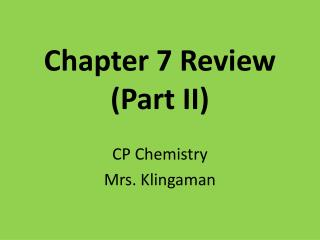# Chapter 7 Review (Part II) - PowerPoint PPT PresentationDownload PresentationChapter 7 Review (Part II)

Chapter 7 Review (Part II)Download Presentation## Chapter 7 Review (Part II)

- - - - - - - - - - - - - - - - - - - - - - - - - - - E N D - - - - - - - - - - - - - - - - - - - - - - - - - - -
##### Presentation Transcript

1. Chapter 7 Review(Part II) CP Chemistry Mrs. Klingaman

2. Q:1 What types of atoms are covalent (aka: molecular) compounds made of?

3. A:1 nonmetals

4. Q:2 True or False: When naming ionic compounds, roman numerals are used to express the charge of the transition metal ion.

5. A:2 True

6. Q:3 What is the prefix used for the number 7?

7. A:3 hepta

8. Q:4 What is the name of the compound CuS?

9. A:4 copper (II) sulfide

10. Q:5 What is the chemical formula for aluminum oxalate?

11. A:5 Al2(C2O4)3

12. Q:6 What is the name of compound N2H4?

13. A:6 dinitrogen tetrahydride

14. Q:7 What are the units for molar mass?

15. A:7 g/mol

16. Q:8 What is the molar mass of lithium phosphate?

17. A:8 Li3PO4 MM = 115.8 g/mol

18. Q:9 What is the % by mass of calcium in Ca(NO3)2?

19. A:9 MM = 164.1 g/mol (40.1/164.1) x 100 = 24.44 % Ca

20. Q:10 Which of the following is not a diatomic molecule: O2, N2, K2, or Br2?

21. A:10 K2 Br-I-N-Cl-H-O-F

22. Q:11 What is Avogadro’s number ? (including proper units)

23. A:11 6.022 x 1023 atoms or molecules /1 mole

24. Q:12 How many mols are there in 1.95 x 1025 molecules of CO2?

25. A:12 1.95 x 1025molec. CO2 1 mol CO2 6.022 x 1023molec. CO2 = 32.38 mols CO2

26. Q:13 What is the mass, in grams, of 0.78 mols of barium carbonate?

27. A:13 BaCO3 0.78 mols BaCO3 197.3 g BaCO3 1 mol BaCO3 = 153.9 g BaCO3

28. Q:14 A sample of sodium hydroxide has a mass of 15.2 grams. How many molecules of sodium hydroxide does it contain?

29. A:14 NaOH 15.2 g NaOH 1 molNaOH 6.022 x 1023molec. NaOH 40 g NaOH 1 molNaOH = 2.29 x 1023molec. NaOH

30. Q:15 Which of the following would be considered an empirical formula? P3O9 , N4H7, or C2H4O2 ?

31. A:15 N4H7

32. Q:16 The stimulant nicotine is found to be 74.03% carbon, 17.27% nitrogen, and 8.70% hydrogen. What is its empirical formula?

33. A:16 C5H7N

34. Q:17 What is the % by mass of oxygen in tin (IV) oxide?

35. A:17 SnO2 MM = 150.7 g/mol (32/150.7) x 100 = 21.23 % O

36. Q:18 What is the mass, in grams, of 2.11 mols of phosphorpustrichloride?

37. A:18 PCl3 2.11 mols PCl3 137.5 g PCl3 1 mol PCl3 = 290.1 g PCl3